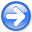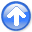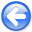Tomographic full waveform inversion and linear modeling of multiple scatteringNext: Numerical 1D example Up: Tomographic Full Waveform Inversion Previous: Tomographic Full Waveform Inversion

The gradient computation of the TFWI objective function has three components. The first component takes into account the dependency of the background wavefield from velocity; it is the same as for the FWI case. The second component is related to the dependency of the approximation of the scattered wavefield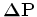from the extended velocity. It is performed by applying the adjoint of the linear operator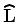. The application of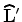to the data residual is accomplished similarly to the FWI gradient by injecting the residual at the receiver location and running backward in time the propagation expressed in equation 17.
The third component takes into account the dependency offrom the velocity at zero time lag; its evaluation is more involved than for the previous two terms but is crucial to the convergence of the inversion towards a velocity model that explain the kinematics in the data. The forward operator can be evaluated as a chain of two operators. The first one relates perturbations in velocity to perturbations in the background wavefield, as expressed by equations 7-8. The second one is computed by forward solving in time equation 17, where the source term is given by the perturbations in the background wavefield, and not by the perturbations in the extended velocity. Consequently this term is zero when the extended velocity perturbations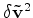are zero, independently from the perturbations in the background wavefield. The adjoint is computed by applying the adjoint of these two operators in reverse order.
Finally, the gradient of the regularization terms depends on the expression of the specific focusing operator. For the choice expressed in equation 23, the computation of the gradient is trivial.Tomographic full waveform inversion and linear modeling of multiple scattering